Point Slope Form and Two Point Form

# Point Slope Form and Two Point Form - Mathematics (Maths) Class 11 - Commerce

C. Standard Equations of Straight Lines

(1) General Form : Any first degree equation of the form Ax + By + C = 0, where A, B, C are constant always represents general equation of a straight line (at least one out of A and B is non zero.)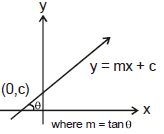(2) Slope - Intercept Form :

y = mx + c

where m = slope of the line = tanθ

c = y intercept

(3) Intercept Form :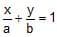x intercept = a

y intercept = b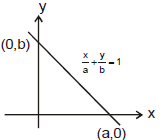(4) Normal Form :

x cos α + y sin α = p ,where α is the angle which the perpendicular to the line makes with the axis of x and ρ is the length of the perpendicular from the origin to the line. p is always positive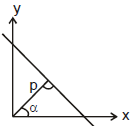(5) Slope Point Form :

Equation : y - y1 = m (x - x1), is the equation of line passing through the point (x1, y1) and having the slope 'm'

(6) Two points Form :

Equation : y - y1 =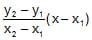, is the equation of line passing through two points (x1, y1) and (x2, y2)

(7) Parametric Form :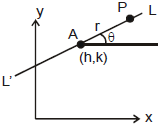To find the equation of a straight line which passes through a given point A(h, k) and makes a given angle θ with the positive direction of the x-axis. P(x, y) is any point on the line LAL'.

Let AP = r, x - h = r cosθ, y - k = r sinθ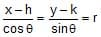is the equation of the straight line LAL'.

Any point on the line will be of the form (h + r cosθ, k + r sinθ), where |r| gives the distance of the point P from the fixed point (h, k)

Note : If point P is taken relatively upward to A then r is positive otherwise negative. If line is parallel to x-axis then for he point right to A, r is positive and for left to A, r is negative.

D. Reduction Of General Equation To Different Standard Forms

(1) Slope - Intercept Form :

To reduce the equation Ax + By + C = 0 to the form y = mx + c

Given equation is Ax+ By + C = 0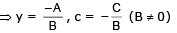Note : Slope of the line Ax + By + c = 0 is -A/B i.e.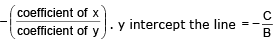(2) Intercept Form :

To reduce the equation Ax + By + C = 0 the form x/a + y/b = 1. This reduction is possible only when C ≠ 0 Given equation is Ax + By = – C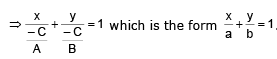Note : Intercept on the x-axis =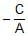, intercept on the y-axis =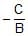. Thus intercept of a straight line on the x-axis can be obtained by putting y = 0 in the equation of the line and then finding the value of x. similarly, intercept on the y-axis can be obtained by putting x = 0 and solving for y.

(3) Normal form :

To reduce the equation Ax + By + C = 0 to the form x cos a + y sin a = p

Given equation is Ax + By + C = 0 or, Ax + By = - C

Case I : When – C > 0, then normal form is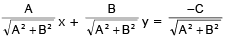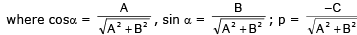Case II : When –C < 0, the write the equation as –Ax – By = C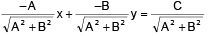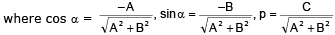Note : In the normal form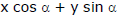= p, p is always taken as positive.

Ex.5 Reduce the line 2x - 3y + 5 = 0, in slope intercept, intercept and normal forms.

Sol.

Slope - Intercept Form :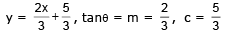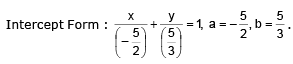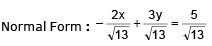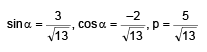Ex.6 Find the equations of the lines which pass through the point (3, 4) and the sum of their respective intercepts on the axes is 14.

Sol.

Let the equation of the line be x/a + y/b = 1 ....(1)

This passes through (3, 4) therefore 3/a + 4/b ....(2)

It is given that a + b = 14   =  b = 14 – a

Putting b = 14 – a in (ii), we get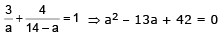⇒ (a – 7) (a – 6) = 0 ⇒  a = 7, 6 ⇒ two such lines are there.

For a = 7, b = 14 – 7 = 7 and for a = 6, a = 14 – 6 = 8

Putting the values of a and b in (i), we get the equations of lines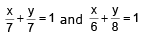or x + y = 7 and 4x + 3y = 24.

Ex.7 A rod of steel is fixed at A (4, 0) and a toy is placed on it at B (0, 4). Now rod is rotated about A through an angle of 15° in clockwise direction, then find the new position of a toy.

Sol.

Let new position of a toy be C.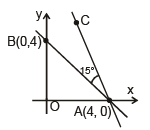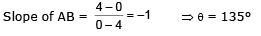Rod is rotated through 15° in clockwise

θnew = 135° - 15° = 120°

AB = 4√2 ⇒  h = 4, k = 0

Hence C = (h + r cos θ, k = r sin θ) = (4 + 4√2 cos 120°, 0 + 4√2 sin 120°) = (4 – 2√2, 2√2 )

Ex.8 If the straight line through the point P(3, 4) makes an angle π/6 with the x-axis and meets the line 12x + 5y + 10 = 0 at Q, find the length of PQ

Sol. The equation of a line passing through P(3, 4) and making an angle = π/6 with the x-axis is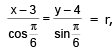where r represents the distance of any point on this line from the given point P(3, 4). The co-ordinates of any point Q on this line are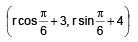If Q lies on 12x +15y + 10 = 0, then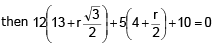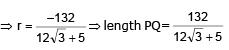Ex.9 A canal is 4 1/2  kms from a place and the shortest route from this place to the cenal is exactly north east. A village is 3 kms north and 4 kms east from the place. Does it lie on canal?

Sol.

Let AB be the canal and O be the given place.
Let L be the foot of perpendicular from O to AB.

Given, OL = 9/2. And ∠AOL = 45°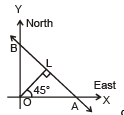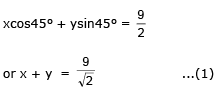Let S be the given village, then S = (4, 3). Putting x = 4 and y = 3 in equation (1), we get 4 + 3  = 9/2 which is not true. Thus the co-ordinates of S doesn't satisfy equation (1) and hence the given village does not lie on the canal.

(4) Position of a point w.r. to a line L : Ax + By + c = 0

(i) If the points P(x1 y1( & Q(x2, y2) lies on the same side of the line Ax + By + C = 0 then the expressions Axj + Byj + C & Ax2 + By2 + C have same sign otherwise if P and Q lies on opposite side then Ax1 + By1 + C and Ax2 + By2 + C will have opposite sign.

(ii) If only one point is given then position of that point is checked w.r. to origin

The document Point Slope Form and Two Point Form | Mathematics (Maths) Class 11 - Commerce is a part of the Commerce Course Mathematics (Maths) Class 11.
All you need of Commerce at this link: Commerce

## FAQs on Point Slope Form and Two Point Form - Mathematics (Maths) Class 11 - Commerce

 1. What is the point-slope form of a linear equation?Ans. The point-slope form of a linear equation is given by y - y1 = m(x - x1), where (x1, y1) are the coordinates of a point on the line and m is the slope of the line.
 2. How do I convert a linear equation from point-slope form to slope-intercept form?Ans. To convert a linear equation from point-slope form to slope-intercept form (y = mx + b), you need to rearrange the equation and solve for y. Start by distributing the slope (m) to the terms within the parentheses, then isolate y by adding y1 to both sides of the equation. Finally, simplify the equation to the form y = mx + b.
 3. Can I use the point-slope form to find the equation of a line given two points?Ans. Yes, you can use the point-slope form to find the equation of a line given two points. Start by calculating the slope using the formula (y2 - y1) / (x2 - x1), where (x1, y1) and (x2, y2) are the coordinates of the two points. Then, choose one of the points and substitute its coordinates along with the calculated slope into the point-slope form equation.
 4. What is the two-point form of a linear equation?Ans. The two-point form of a linear equation is given by (y - y1) / (y2 - y1) = (x - x1) / (x2 - x1), where (x1, y1) and (x2, y2) are the coordinates of two different points on the line. This form allows you to find the equation of a line directly using two given points.
 5. How do I convert a linear equation from two-point form to slope-intercept form?Ans. To convert a linear equation from two-point form to slope-intercept form (y = mx + b), you need to rearrange the equation and solve for y. Start by cross-multiplying to eliminate the denominators. Then, simplify the equation to the form y = mx + b by distributing and rearranging the terms. The resulting equation will be in slope-intercept form.

## Mathematics (Maths) Class 11

157 videos|210 docs|132 tests

## Mathematics (Maths) Class 11

157 videos|210 docs|132 tests
Signup to see your scores go up within 7 days! Learn & Practice with 1000+ FREE Notes, Videos & Tests.
10M+ students study on EduRev
Track your progress, build streaks, highlight & save important lessons and more!(Scan QR code)
Related Searches

,

,

,

,

,

,

,

,

,

,

,

,

,

,

,

,

,

,

,

,

,

;